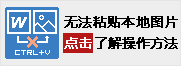•  您的网站试用资格已过期。

# 【信息奥赛】0.1原码反码补码[练习答案]

11-6=5

[注：我们可以设置5位二进制数，最高位为符号位]

11=1*23+0*22+1*21+1*20=10112

6=0*23+1*22+1*21+0*20=01102

11=010112    -6=101102(最高位为符号位)

A=11=010112     B=-6=101102

A[]=010112            B[]= 101102

A[]=010112            B[]= 110012

（注：负数反码，符号位为1，其余各数值与原码相反）

A[]=010112            B[] = 110102

（注：负数补码，符号位为1，数值部分值为反码数值+1

(1)比大小，10112>01102 ，则最高位符号位为“0

(2)(3)结果为00101

（注：将结果转为真值就是+101，转为十制进就是5

(1)(2)如果最高位产生进制（这儿第6位为1），则进位1去掉，数值位+1当然，没有产生进制，数值位就不必+1！！！

00100+1=00101

（注：将结果转为真值就是+101，转为十制进就是5

(1) 注：补码，可以忽视最高位进位！(2)将结果转为真值就是+101，转为十制进就是5